# What Is An Equation With No Solution? (3 Key Ideas)

In mathematics, we want to solve an equation to find the values of variables that make it true.  However, this is not always possible.

So, what is an equation with no solution?  An equation with no solution is never true, regardless of which values we choose for the variables in the equation. We can have linear equations, absolute value equations, radical equations, and quadratic equations with no solution. Higher order polynomial equations can also have no solution.

Of course, there may be more than one way to show that an equation has no solution.  For example, we can use algebra or draw a graph to show that no solutions exist.

In this article, we’ll talk about various equations with no solution.  We’ll also look at some examples to make the concept clear.

Let’s get started.

## What Is An Equation With No Solution?

An equation with no solution is one that is never true, regardless of which values we choose for the variables in the equation.

An equation with no real solution is one that is never true, regardless of which real numbers we choose for the variables in the equation.  However, an equation with no real solution may still have complex solutions (as we will see later).

Often, an equation with no solution comes from trying to find the intersection points of two curves that never meet.

For example, consider these two functions:

• y = x3
• y = x3 + 1

If we use algebra to find out where they intersect, we get:

• y = y
• x3 = x3 + 1
• 0 = 1

This is never true, regardless of which value we choose for x.  So, the equation has no solution.

We can see this visually in the graph below.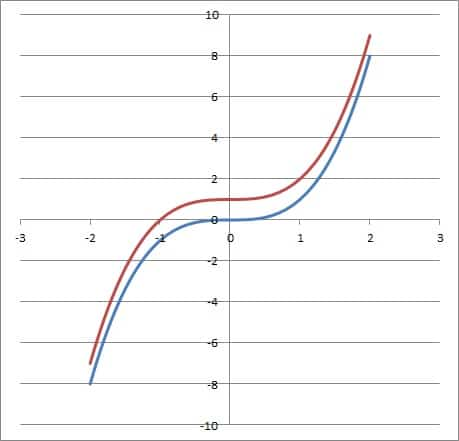The graphs of y = x3 and y = x3 + 1. Note that the never intersect, since the second curve (red) is always one unit above the first curve (blue).

We can find a polynomial equation of any degree with no solution, but there are other cases as well.  Some of the most common one are:

• Linear Equations With No Solution
• Absolute Value Equations With No Solution
• Radical Equations With No Solution
• Quadratic Equations With No Solution
• Quadratic Equations With No Real Solution

Let’s take a look at each of these situations in turn, along with some examples to illustrate the idea.  We’ll start with linear equations with no solution.

## Linear Equations With No Solution

A linear equation can have no solution it if reduces to a statement that is not true.  Generally, this will be a statement that a constant value is equal to a different constant value (for example, 2 = 5).

Often, a linear equation with no solution occurs if we try to find the intersection of two lines that never intersect (that is, the intersection of two parallel lines).

Let’s look at some examples.

### Example 1: Linear Equation With No Solution

Consider the linear equation

• 5x + 2 = 5x + 6

There are only linear and constant terms, so both sides of the equation are linear.

However, when we try to solve, we get:

• 5x + 2 = 5x + 6
• 5x + 2 – 5x = 5x + 6 – 5x  [add -5x to both sides]
• 2 = 6

Since 2 = 6 is always false, we know that this linear equation has no solution.  No matter which value of x we choose, the original equation will never be true.

You can see from the graph below that the two lines y = 5x + 2 and y = 5x + 6 never intersect.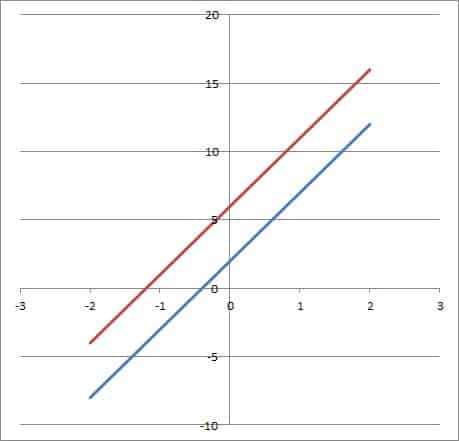The graphs of y = 5x + 2 and y = 5x + 6. They never intersect, since the upper line (red) is always 4 units above the lower line (blue).

### Example 2: Linear Equation With No Solution

Consider the linear equation

• 4x – 3x – 2 = 7x – 6x + 6

There are only linear and constant terms, so both sides of the equation are linear.

However, when we try to solve, we get:

• 4x – 3x – 2 = 7x – 6x + 6
• x – 2 = x + 6  [combine like terms on both sides]
• x – 2 – x = x + 6 – x
• -2 = 6  [subtract x from both sides]

Since -2 = 6 is always false, we know that this equation has no solution.  No matter which value of x we choose, the original equation will never be true.

You can see from the graph below that the two lines y = x – 2 and y = x + 6 never intersect.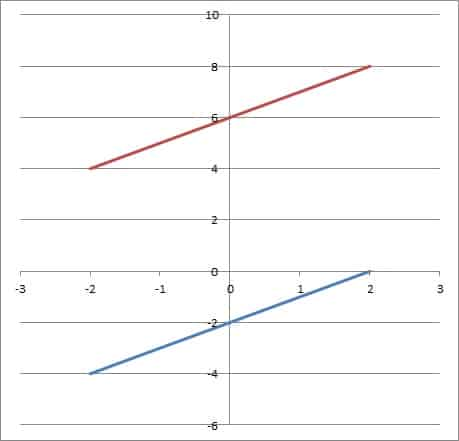The graphs of y = x – 2 and y = x + 6. They never intersect, since the upper line (red) is always 8 units above the lower line (blue).

## Absolute Value Equations With No Solution

An absolute value equation can have no solution it if reduces to a statement that is not true.  Generally, this will be a statement that an absolute value is equal to a negative number (for example, |x| = -4).

Remember that an absolute value cannot be negative, but the solutions can be.  (If you need a refresher, check out my article here.)

Often, an absolute value equation with no solution occurs if we try to find the intersection of an absolute value function and a horizontal line below the x-axis.

Let’s look at some examples.

### Example 1: Absolute Value Equation With No Solution

Consider the absolute value equation

• |x – 2| = -5

We can already tell that there is no solution, since the absolute value on the left side is always nonnegative (positive or zero), and the number on the right side is negative.

Since an absolute value is never negative, we know that this equation has no solution.  No matter which value of x we choose, the original equation will never be true.

You can see from the graph below that the two functions y = |x – 2| and y = -5 never intersect.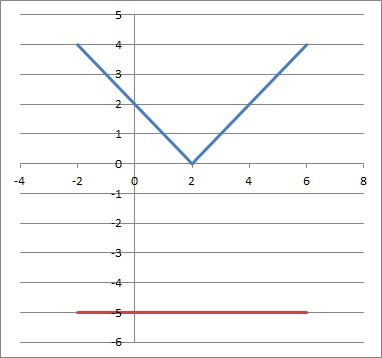The graphs of y = |x – 2| and y = -5. They never intersect, since the upper line (blue) is always below the lower line (red).

### Example 2: Absolute Value Equation With No Solution

Consider the absolute value equation

• |x| = x – 1

One way to tell that there is no solution is to rewrite the absolute value on the left as a piecewise function.  Instead of |x|, we can write:

• -x, for x < 0
• x, for x >= 0

First, we search for negative solutions (x < 0):

• -x = x – 1
• 0 = 2x – 1
• 1 = 2x
• ½ = x

However, this is not valid, since x = ½ is not negative.

Now, we search for nonnegative solutions (x >= 0):

• x = x – 1
• 0 =  – 1

This is false, so it has no solution.  No matter which value of x we choose, the original equation will never be true.

You can also see from the graph below that the two functions y = |x| and y = x – 1 never intersect.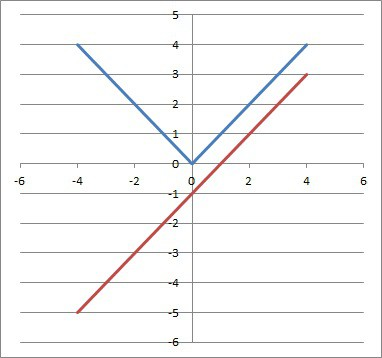The graphs of y = |x| and y = x – 1. They never intersect, since the upper line (blue) is always below the lower line (red).

## Radical Equations With No Solution

A radical equation can have no solution it if reduces to a statement that is not true.  Generally, this will happen after we raise both sides of an equation to a power to eliminate the radical (for example, √(x2 +2x) = x + 1).

Often, a radical equation with no solution occurs if we try to find the intersection of a radical function and a function that is always negative (below the x-axis).

Let’s look at some examples.

### Example 1: Radical Equation With No Solution

• √(x2 +2x) = x + 1

Since the radical (a square root) has an index of 2, the approach for solving is to square both sides (raise both sides to the power of 2):

• √(x2 +2x) = x + 1
• (√(x2 +2x))2 = (x + 1)2  [square both sides]
• x2 + 2x = x2 + 2x + 1  [FOIL the right side]
• 0 = 1 [subtract x2 + 2x from both sides]

Since 0 = 1 is always false, we know that this equation has no solution.  No matter which value of x we choose, the original equation will never be true.

You can see from the graph below that the two curves y = √(x2 +2x) and y = x + 1 never intersect.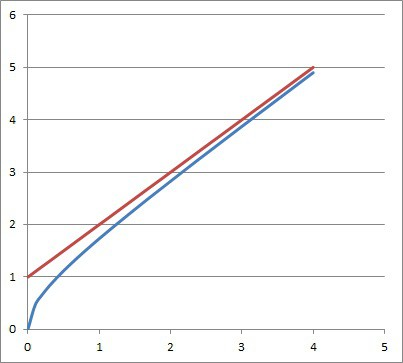The graphs of y = √(x2 +2x) and y = x + 1. They never intersect, since the upper line (red) is always above the lower line (blue).

### Example 2: Radical Equation With No Solution

• √(x4 + 8x2) = x2 + 4

Since the radical (a square root) has an index of 2, the approach for solving is to square both sides (raise both sides to the power of 2):

• √( x4 + 8x2) = x2 + 4
• (√(x4 + 8x2))2 = (x2 + 4)2  [square both sides]
• x4 + 8x2 = x4 + 8x2 + 16  [FOIL the right side]
• 0 = 16 [subtract x4 + 8x2 from both sides]

Since 0 = 16 is always false, we know that this equation has no solution.  No matter which value of x we choose, the original equation will never be true.

You can see from the graph below that the two curves y = √(x4 + 8x2) and y = x2 + 4 never intersect.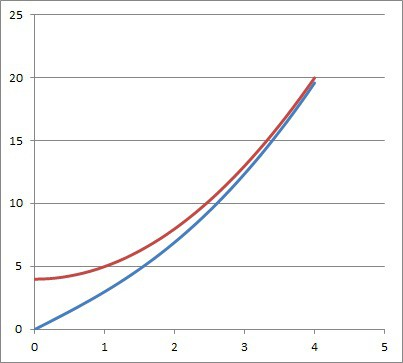The graphs of y = √(x4 + 8x2) and y = x2 + 4. They never intersect, since the upper line (red) is always above the lower line (blue).

## Quadratic Equations With No Solution

A quadratic equation can have no solution it if reduces to a statement that is not true.  Generally, this will be a statement that a constant value is equal to a different constant value (for example, 2 = 5).

Often, a quadratic equation with no solution occurs if we try to find the intersection of two parabolas that never intersect (for example, the intersection of two parabolas with the same values of a and b, but different values of c).

Let’s look at some examples.

### Example 1: Quadratic Equation With No Solution

• x2 + 2 = x2 + 5

Both sides of the equation are quadratic, so we have a quadratic equation.

However, when we try to solve, we get:

• x2 + 2 = x2 + 5
• 2 = 5  [subtract x2 from both sides]

Since 2 = 5 is always false, we know that this equation has no solution.  No matter which value of x we choose, the original equation will never be true.

You can see from the graph below that the two parabolas y = x2 + 2 and y = x2 + 5 never intersect.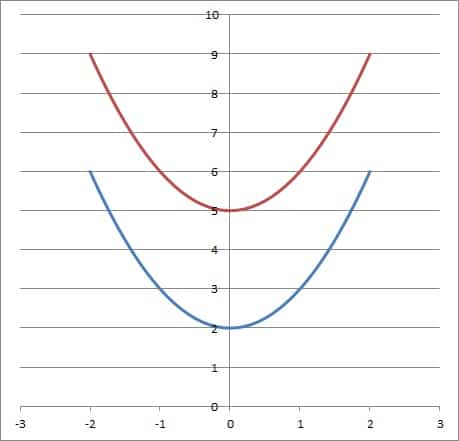The graphs of y = x2 + 2 and y = x2 + 5. They never intersect, since the upper line (red) is always 3 units above the lower line (blue).

### Example 2: Quadratic Equation With No Solution

• (x + 2)2 = x2 + 4x – 5

Both sides of the equation are quadratic, so we have a quadratic equation.

However, when we try to solve, we get:

• (x + 2)2 = x2 + 4x – 5
• x2 + 4x + 4 = x2 + 4x – 5  [FOIL the left side]
• 4 = -5  [subtract x2 + 4x from both sides]

Since 4 = -5 is always false, we know that this equation has no solution.  No matter which value of x we choose, the original equation will never be true.

You can see from the graph below that the two parabolas y = (x + 2)2 and y = x2 + 4x – 5 never intersect.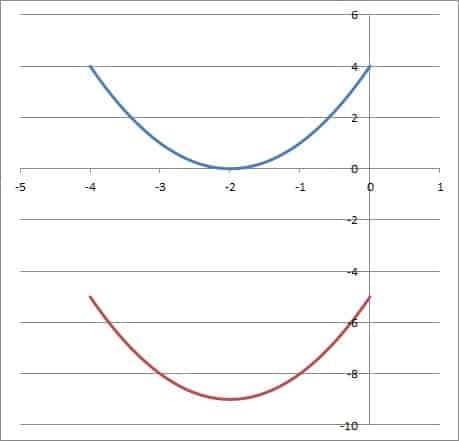The graphs of y = (x + 2)2 and y = x2 + 4x – 5. They never intersect, since the upper line (blue) is always 9 units above the lower line (red).

## Quadratic Equations With No Real Solution

A quadratic equation can have no real solution if the discriminant is negative (that is, b2 – 4ac < 0, or b2 < 4ac).

The graph of a quadratic with no real solution is a parabola that never touches the x-axis (that is, it is always above or always below the x-axis).

A quadratic equation with no real solution has two complex solutions, which are complex conjugate roots of the form a + bi and a – bi.

Let’s take a look at some examples.

### Example 1: Quadratic Equation With No Real Solution

• 2x2 + 4 = -x2 – 5

Both sides of the equation are quadratic, so we have a quadratic equation.

However, when we try to solve, we get:

• 2x2 + 4 = -x2 – 5
• 3x2 = -9  [move and combine like terms]
• x2 = -3
• x = √(-3)
• x = 3i

Since x = 3i is imaginary, we know that there is no real solution to this equation.

You can see from the graph below that the two parabolas y = 2x2 + 4 and y = -x2 – 5 never intersect.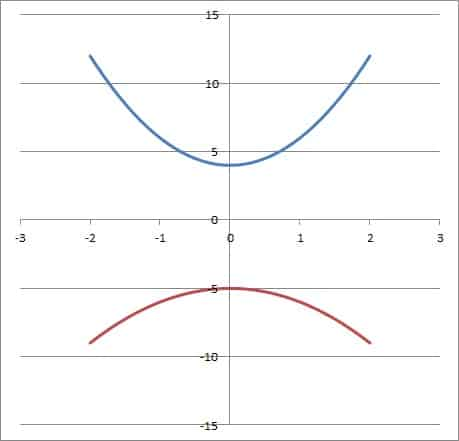The graphs of y = 2x2 + 4 and y = -x2 – 5. They never intersect, since the upper line (blue) is always above the lower line (red).

### Example 2: Quadratic Equation With No Real Solution

• 6x2 + x + 11 = 0

This is a quadratic equation with a = 6, b = 1, and c = 11.

Calculating the discriminant, we get:

• b2 – 4ac
• =12 – 4(6)(11)
• =1 – 264
• =-263

Since the discriminant -263 is negative, we know that there is no real solution to this quadratic equation.  Instead, the two solutions are complex conjugate roots.

You can see from the graph below that the parabola from the quadratic function y = 6x2 + x + 11 never intersects the x-axis.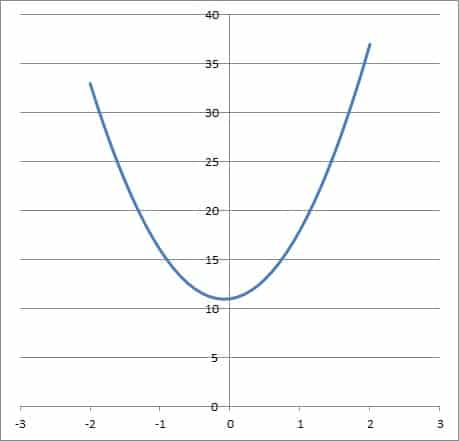The graph of y = 6x2 + x + 11 never touches the x-axis, so the corresponding quadratic equation has no real solution.

## Conclusion

Now you know about some scenarios where you might see an equation with no solution.  You also know what to look for (like an absolute value equal to a negative number) to tell that there is no solution.

You might also want to read my article on equations with one solution.

You can learn about the difference between expressions and equations here.